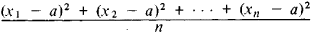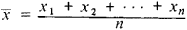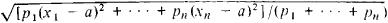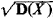# Root-Mean-Square Deviation

Also found in: Acronyms, Wikipedia.

## root-mean-square deviation

[¦rüt ¦mēn ¦skwer ‚der·ə′vā·shən]
(statistics)
The square root of the sum of squared deviations from the mean divided by the number of observations in the sample.
McGraw-Hill Dictionary of Scientific & Technical Terms, 6E, Copyright © 2003 by The McGraw-Hill Companies, Inc.
The following article is from The Great Soviet Encyclopedia (1979). It might be outdated or ideologically biased.

## Root-Mean-Square Deviation

The root-mean-square (rms) deviation of the quantities x1, x2, …, xn from a is the square root of the expressionThe rms deviation has its least value when a = , where is the arithmetic mean of the quantities x1, x2, … xnIn this case the rms deviation may serve as a measure of the dispersion of the system of quantities x1, x2, … xn. The more general concept of weighted rms deviationis also used; p1, … pn in this case are called the weights corresponding to the quantities x1, … xn. The weighted rms deviation attains its least value when a is equal to the weighted mean:

(p1x1 + ..... + pnxn)/(p1 + ... + pn)

In probability theory the weighted rms deviation σx of a random variable X (from its mathe matical expectation) is the square root of the variance, and is called the standard deviation of X.

The standard deviation is used as a measure of the quality of statistical estimates and in this case is called the standard error.